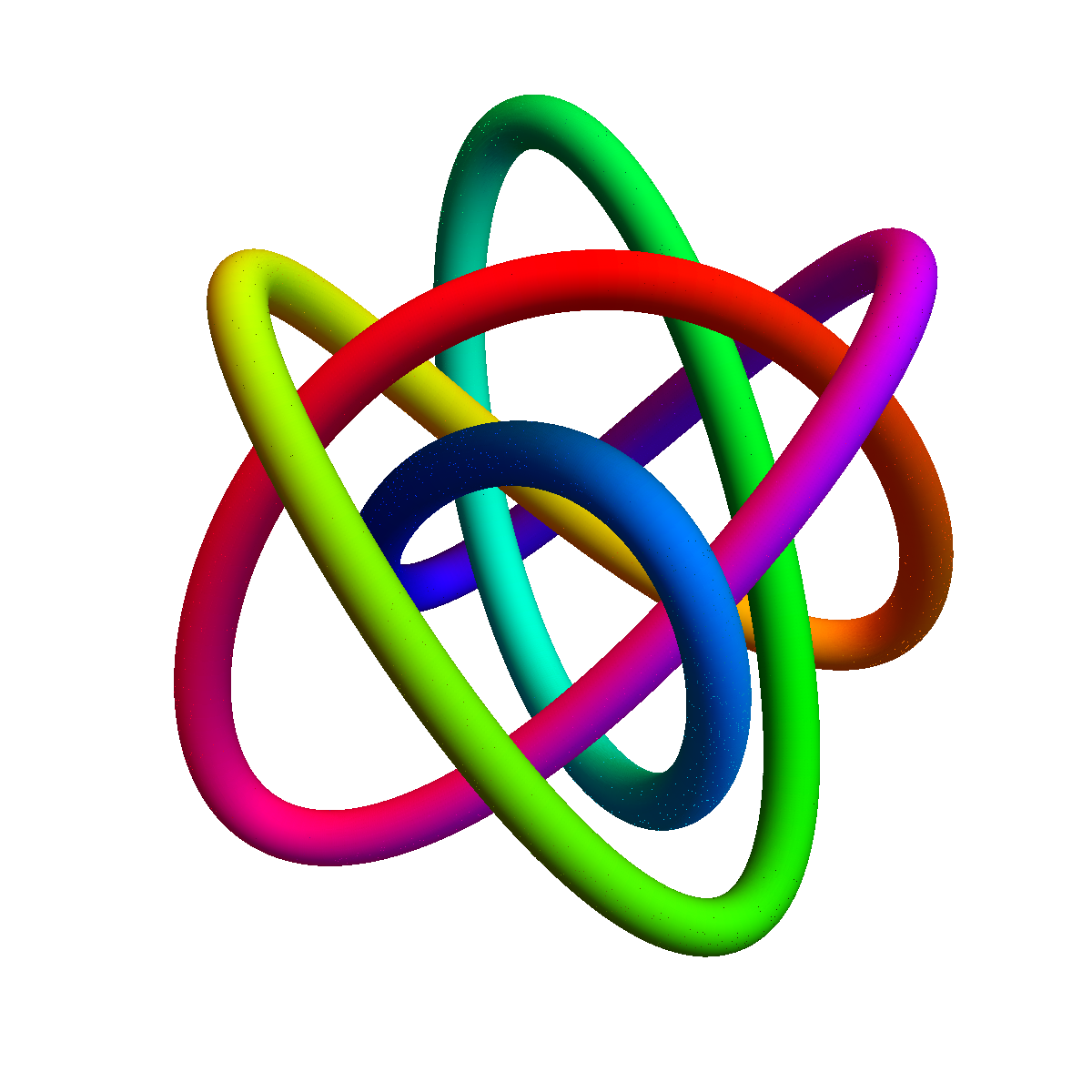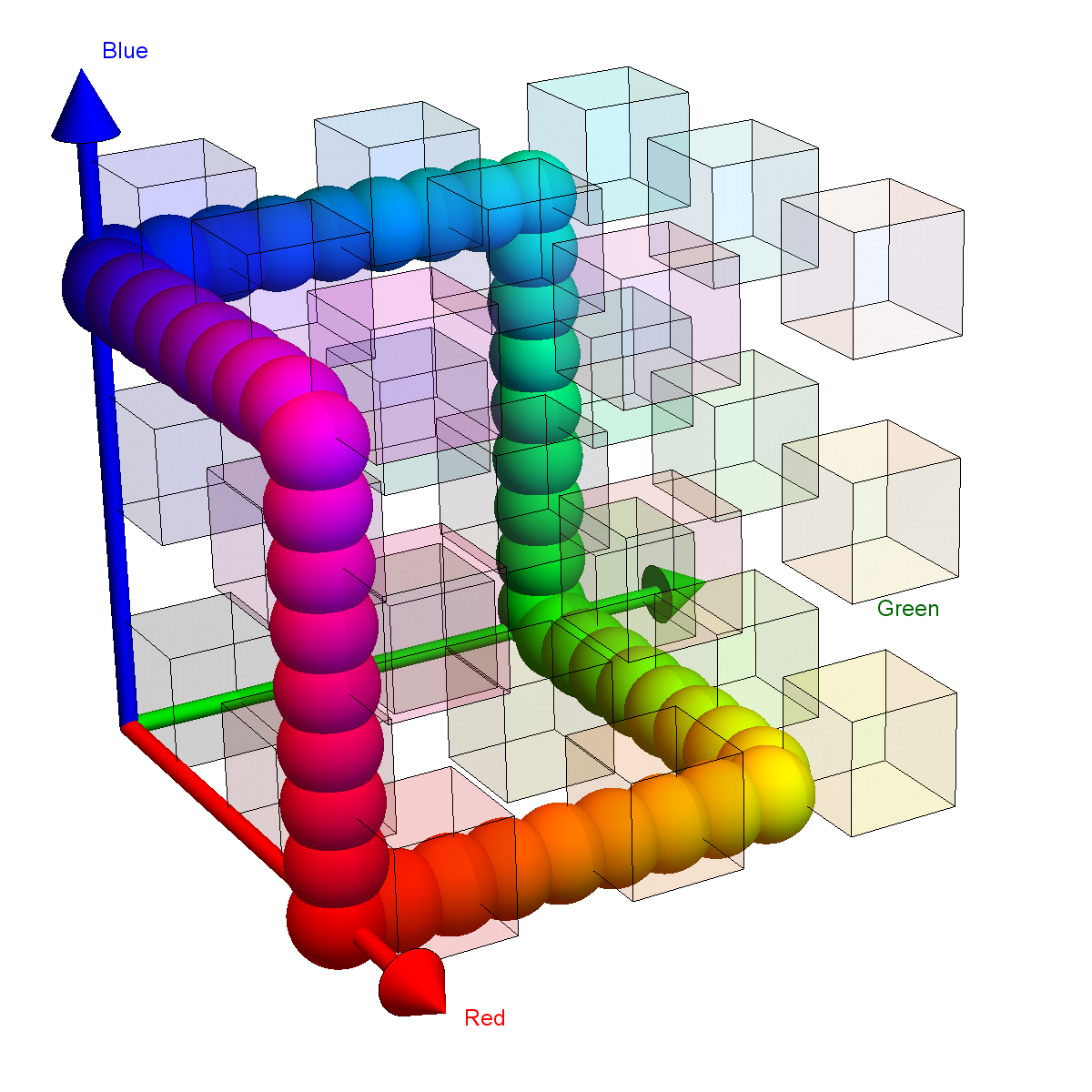# Untie a knot in four dimensions

This is a new version of the animation done for an exhibit in 2006. But it is done for the knot which appeared in the homework. It is a Lissajoux knot. We see again that if we use color as the fourth dimension and make sure that during the cross over we have different colors at the points, then we can untie the knot. Below you see the picture of the knot and also the picture of the hue path in the red-green-blue color space. Every value hue(t) is a vector hue(t)=[r(t),g(t),b(t)] in the RGB color space. The picture shows that it is a closed unknotted path. If we have a curve r(t), then we essentially assigned the color hue(t) to the point t. In other words, we actually see a path even in a 6 dimensional space as we have now [x(t),y(t),z(t),r(t),g(t),b(t)]. The hue function allows us know to get a path in four dimensions which is [x(t),y(t),z(t),h(t)]. Now we can deform the path so that it becomes the trivial circle [ cos(t),sin(t),0,h(t)] which then could be uni-colored [cos(t),sin(t),0,0]. It is the ``unknot". Any knot which can be deformed into an unknot (without every self-intersecting) is called a trivial knot. The Lissajoux knot seen here is not trivial in R3 but it is in R4. The four dimensional space is just much bigger. Some additional tid-bits: also in 2 dimensions, we can not have knots as any closed curve without self-intersection is a trivial curve. This is the Jordan curve theorem which is not that easy to prove. The engineers who first came up with the Hue path, have defined an only piece-wise continuously differentiable path. They wanted to go through all the major colors like red, green, blue, cyan, magenta or yellow or pink, purple and orange. We could easily design other color paths some of which are smooth or not closed paths. You find many example lists on the web (just google "color map"). One can draw color curves also in the color wheel (like HSV Color circle) which roughly means taking the Hue color H as the polar angle from 0 to 2pi and then let the radius be a saturation parameter S. It can also be drawn in 3 dimensions, where one has then the HSV cylinder, the third coordinate is then the brightness value (abbreviated simply V=value or L=lightness which is the HSL cylinder). You find some formulas here. The point is that the color models in computer graphics alone require quite a bit of vector analysis and involve various coordinate systems.

Direct Media Links: Webm, Ogg Ipod ;